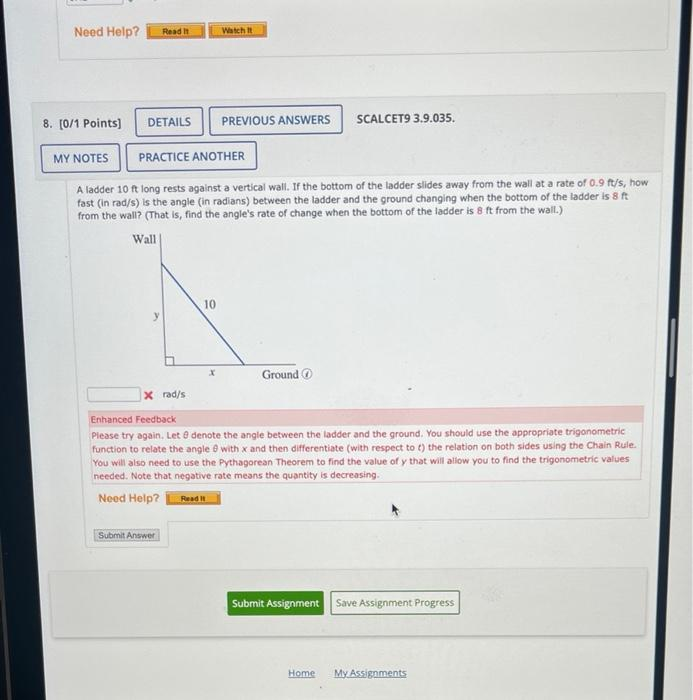# (Solved): A ladder 10ft long rests against a vertical wall. If the bottom of the ladder slides away from the ...A ladder long rests against a vertical wall. If the bottom of the ladder slides away from the wall at a rate of , how fast (in rad/s) is the angle (in radians) between the ladder and the ground changing when the bottom of the ladder is from the wall? (That is, find the angle's rate of change when the bottom of the ladder is from the wall.) Enhanced Feedback Please try again. Let denote the angle between the ladder and the ground. You should use the appropriate trigonometric function to relate the angle with and then differentiate (with respect to ) the relation on both sides using the Chain Rule. You will also need to use the Pythagorean Theorem to find the value of that will allow you to find the trigonometric values needed. Note that negative rate means the quantity is decreasing.

We have an Answer from Expert